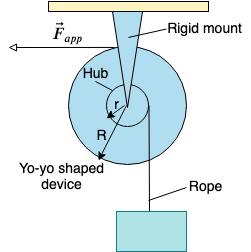# A yo-yo-shaped device mounted on a horizontal frictionless axis is used to lift a 30 kg box as...

## Question:

A yo-yo-shaped device mounted on a horizontal frictionless axis is used to lift a 30 kg box as shown. The outer radius R of the device is 0.50 m, and the radius r of the hub is 0.20 m. When a constant horizontal force of magnitude 140 N is applied to a rope wrapped around the outside of the device, the box, which is suspended from a rope wrapped around the hub, has an upward acceleration of magnitude 0.80 m/s2. What is the rotational inertia of the device about its axis of rotation and what is the tension in the rope supporting the box?## Torque:

Torque is the product of the force and the lever arm. Its presence signifies angular acceleration. The torque, for a rigid body, is equal to the product of the moment of inertia and the angular acceleration.

Become a Study.com member to unlock this answer! Create your account

The torque is given as:

{eq}\tau = I\alpha {/eq}

The torque can be solve by:

{eq}\tau = F_{app}R \\ \tau = (140)(0.50) \\ \tau = 70 \ Nm {/eq}

...Torque: Concept, Equation & Example

from

Chapter 7 / Lesson 4
13K

After watching this lesson, you will be able to explain what torque is conceptually and mathematically and use both the equation and Newton's First Law for rotation to solve problems involving torque. A short quiz will follow.